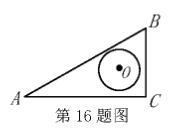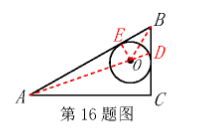【答案】 $2 \sqrt{7}+1$

【解析】 解：当 $\mathrm{BO}$ 为 $\angle \mathrm{B}$ 角平分线, 且 $\mathrm{BA} 、 \mathrm{BC}$ 均为 $\odot 0$ 的切线时, 连接 $\mathrm{AO}$ 并延长交 $\odot 0$ 于 点 D, 此时 $A D$ 即为点 $A$ 到 $\odot 0$ 上的点的距离的最大值, $\because \angle C=90^{\circ}, A C=6, B C=2 \sqrt{3}$, $\therefore \tan \angle \mathrm{B}=\frac{\mathrm{AC}}{\mathrm{BC}}=\sqrt{3}, \therefore \angle \mathrm{B}=60^{\circ}, \angle \mathrm{A}=30^{\circ}$, 过 0 作 $\mathrm{OE} \perp \mathrm{AB}$ 于 $\mathrm{E}$, 则 $\mathrm{E}$ 为切点, $\angle$ $\mathrm{OBE}=30^{\circ}, \quad \therefore \mathrm{OB}=20 \mathrm{E}=2, \quad \mathrm{BE}=\sqrt{3} \mathrm{OE}=\sqrt{3}, \quad \because \mathrm{AB}=2 \mathrm{BC}=4 \sqrt{3}, \quad \therefore \mathrm{AE}=\mathrm{AB}-\mathrm{BE}=4 \sqrt{3}-\sqrt{3}=3 \sqrt{3}$, $\therefore O A=\sqrt{O E^2+A E^2}=\sqrt{1+27}=2 \sqrt{7}, \quad A D=O A+O D=2 \sqrt{7}+1$, 即点 $A$ 到 $\odot 0$ 上的点的距离的 最大值为 $2 \sqrt{7}+1$.